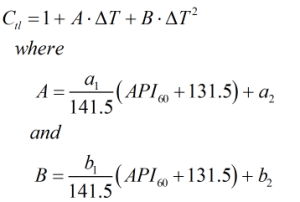# C198 – ASTM-IP Table 6:1952 – Volume Reduction to 60°F

## Description

This calculates the API gravity at an alternate temperature via a temperature correction factor from API gravity at 60°F and the alternate temperature.

Report on the Development, Construction, Calculation and Preparation of the ASTM-IP Petroleum Measurement Tables – ASTM D 1250; IP 200 (1960)

ASTM-IP Petroleum Measurement Tables – ASTM D 1250; IP 200 (1952)

Kelton calculation reference C198

FLOCALC calculation reference F084

## Options

### Calculation Method

• From algorithm
• From Table

This option is used to select whether the Ctl is calculated from an algorithm using the formulae as outlined below or interpolated from the printed values in Petroleum Measurement Table 6.

### Precision

• Full
• Rounding

If rounding is selected the output is rounded to 4 decimal places as per the table otherwise values are displayed to full precision.

## Calculation

### Temperature Correction

The temperature correction is calculated by :Where ΔT = Difference in temperature compared to base temperature in °F API60 = API gravity at 60°F a1 = Constant from Table III a2 = Constant from Table III b1 = Constant from Table III b2 = Constant from Table III

Back